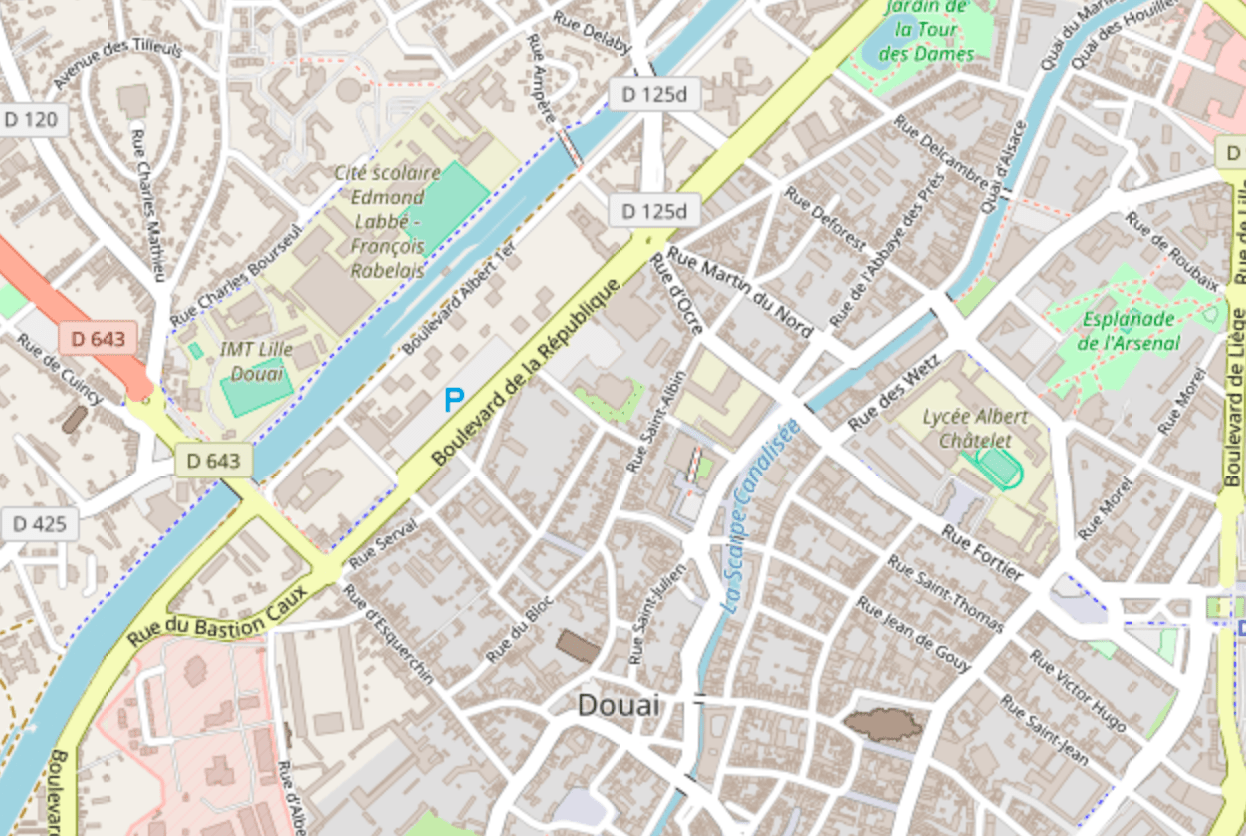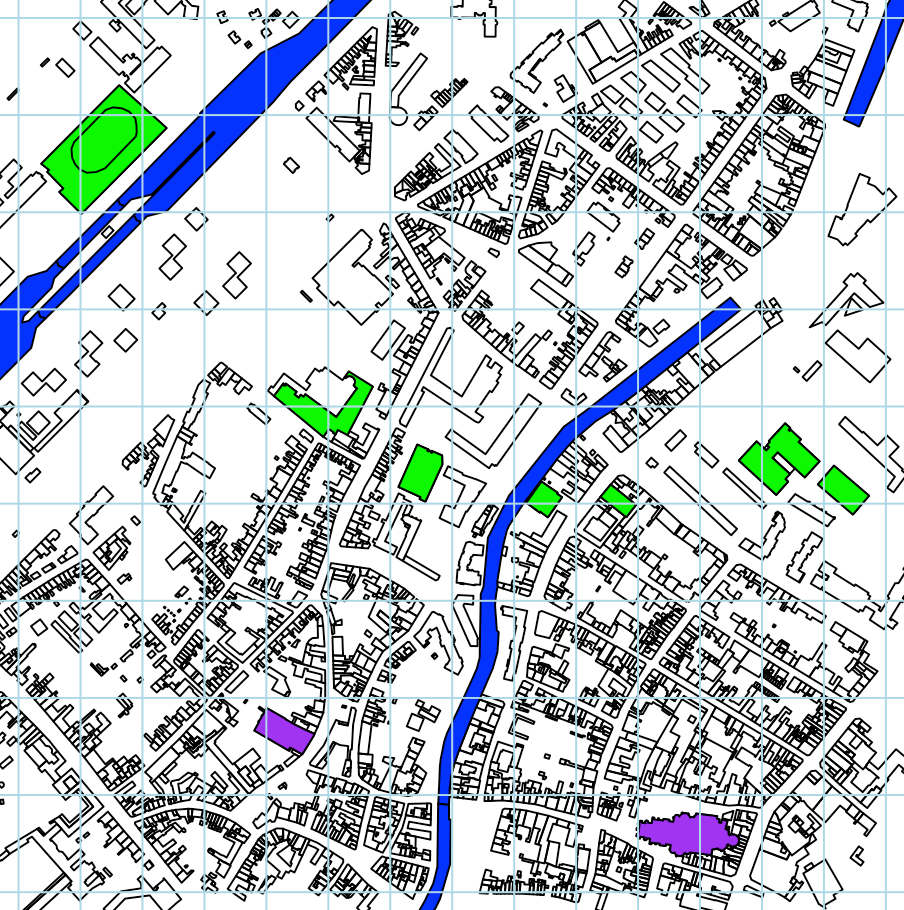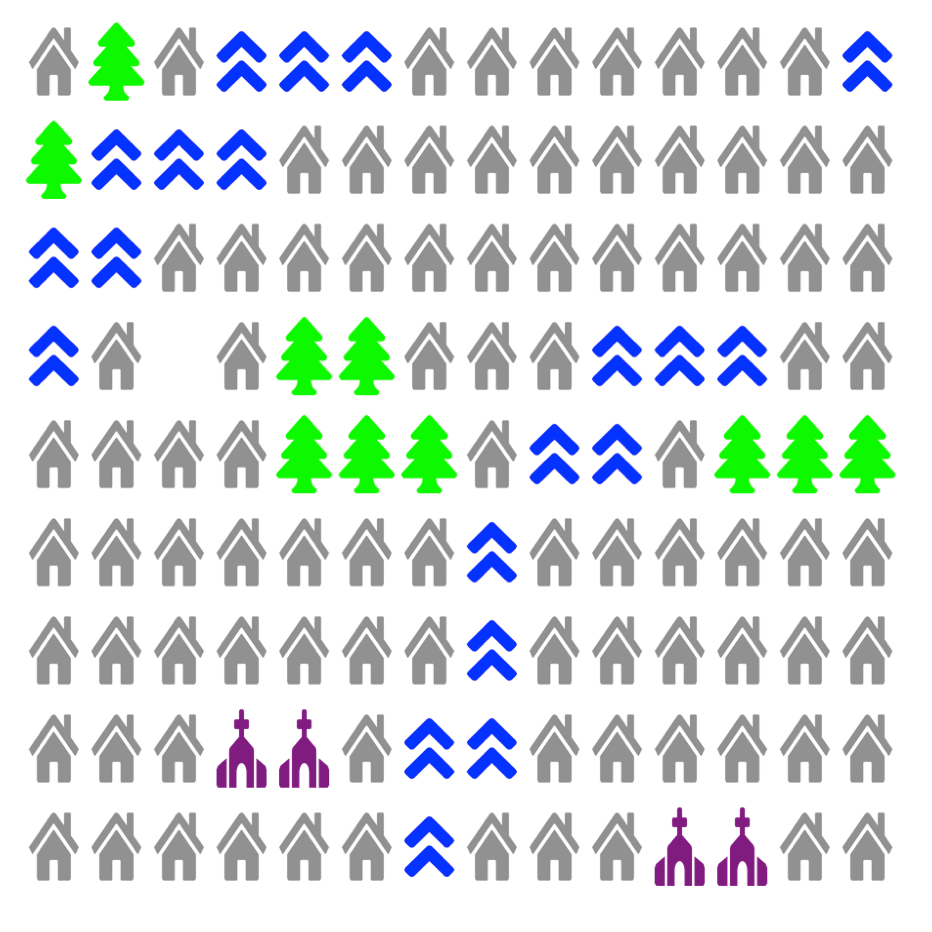Want to share your content on R-bloggers? click here if you have a blog, or here if you don't.

Yesterday evening, I was walking in Budapest, and I saw some nice map that was some sort of Otto Neurath style. It was hand-made but I thought it should be possible to do it in R, automatically.

A few years ago, Baptiste Coulmont published a nice blog post on the package osmar, that can be used to import OpenStreetMap objects (polygons, lines, etc) in R. We can start from there. More precisely, consider the city of Douai, in France,The code to read information from OpenStreetMap is the following

```library(osmar)
src <- osmsource_api()
bb <- center_bbox(3.07758808135,50.37404355, 1000, 1000)
ua <- get_osm(bb, source = src)```

We can extract a lot of things, like buildings, parks, churches, roads, etc. There are two kinds of objects so we will use two functions

```listek = function(vc,type="polygons"){
nat_ids <- find(ua, way(tags(k %in% vc)))
nat_ids <- find_down(ua, way(nat_ids))
nat <- subset(ua, ids = nat_ids)
nat_poly <- as_sp(nat, type)}

listev = function(vc,type="polygons"){
nat_ids <- find(ua, way(tags(v %in% vc)))
nat_ids <- find_down(ua, way(nat_ids))
nat <- subset(ua, ids = nat_ids)
nat_poly <- as_sp(nat, type)}```

For instance to get rivers, use

`W=listek(c("waterway"))`

and to get buildings

`M=listek(c("building"))`

We can also get churches

`C=listev(c("church","chapel"))`

but also train stations, airports, universities, hospitals, etc. It is also possible to get streets, or roads

```H1=listek(c("highway"),"lines")
H2=listev(c("residential","pedestrian","secondary","tertiary"),"lines")```

but it will be more difficult to use afterwards, so let’s forget about those.

We can check that we have everything we need

```plot(M)Now, let us consider a rectangular grid. If there is a river in a cell, I want a river. If there is a church, I want a church, etc. Since there will be one (and only one) picture per cell, there will be priorities. But first we have to check intersections with polygons, between our grid, and the OpenStreetMap polygons.

```library(sp)
library(raster)
library(rgdal)
library(rgeos)
library(maptools)
identification = function(xy,h,PLG){
b=data.frame(x=rep(c(xy-h,xy+h),each=2),
y=c(c(xy-h,xy+h,xy+h,xy-h)))
pb1=Polygon(b)
Pb1 = list(Polygons(list(pb1), ID=1))
SPb1 = SpatialPolygons(Pb1, proj4string = CRS("+proj=longlat +ellps=WGS84 +datum=WGS84 +no_defs +towgs84=0,0,0"))
return(gIntersection(SPb1,UC))
}```

and then, we identify, as follows

```whichidtf = function(xy,h){
h=.7*h
label="EMPTY"
if(!is.null(identification(xy,h,M))) label="HOUSE"
if(!is.null(identification(xy,h,P))) label="PARK"
if(!is.null(identification(xy,h,W))) label="WATER"
if(!is.null(identification(xy,h,U))) label="UNIVERSITY"
if(!is.null(identification(xy,h,C))) label="CHURCH"
return(label)
}```

Let is use colored rectangle to make sure it works

```nx=length(vx)
vx=as.numeric((vx[2:nx]+vx[1:(nx-1)])/2)
ny=length(vy)
vy=as.numeric((vy[2:ny]+vy[1:(ny-1)])/2)
plot(M,border="white")
for(i in 1:(nx-1)){
for(j in 1:(ny-1)){
lb=whichidtf(c(vx[i],vy[j]),h)
if(lb=="HOUSE") rect(vx[i]-h,vy[j]-h,vx[i]+h,vy[j]+h,col="grey")
if(lb=="PARK") rect(vx[i]-h,vy[j]-h,vx[i]+h,vy[j]+h,col="green")
if(lb=="WATER") rect(vx[i]-h,vy[j]-h,vx[i]+h,vy[j]+h,col="blue")
if(lb=="CHURCH") rect(vx[i]-h,vy[j]-h,vx[i]+h,vy[j]+h,col="purple")
}}```As a first start, we us agree that it works. To use pics, I did borrow them from https://fontawesome.com/. For instance, we can have a tree

``` library(png)
library(grid)

Unfortunatly, the color is not good (it is black), but that’s easy to fix using the RGB decomposition of that package

``` rev_tree=tree
rev_tree[,,2]=tree[,,4]```

We can do the same for houses, churches and water actually

``` download.file("https://f.hypotheses.org/wp-content/blogs.dir/253/files/2018/05/angle-double-up.png","angle-double-up.png")
rev_water=water
rev_water[,,3]=water[,,4]
rev_home=home
rev_home[,,4]=home[,,4]*.5
rev_church=church
rev_church[,,1]=church[,,4]*.5
rev_church[,,3]=church[,,4]*.5```

and that’s almost it. We can then add it on the map

``` plot(M,border="white")
for(i in 1:(nx-1)){
for(j in 1:(ny-1)){
lb=whichidtf(c(vx[i],vy[j]),h)
if(lb=="HOUSE")  rasterImage(rev_home,vx[i]-h*.8,vy[j]-h*.8,vx[i]+h*.8,vy[j]+h*.8)
if(lb=="PARK") rasterImage(rev_tree,vx[i]-h*.9,vy[j]-h*.8,vx[i]+h*.9,vy[j]+h*.8)
if(lb=="WATER") rasterImage(rev_water,vx[i]-h*.8,vy[j]-h*.8,vx[i]+h*.8,vy[j]+h*.8)
if(lb=="CHURCH") rasterImage(rev_church,vx[i]-h*.8,vy[j]-h*.8,vx[i]+h*.8,vy[j]+h*.8)
}}```Nice, isn’t it? (as least as a first draft, done during the lunch break of the R conference in Budapest, today).